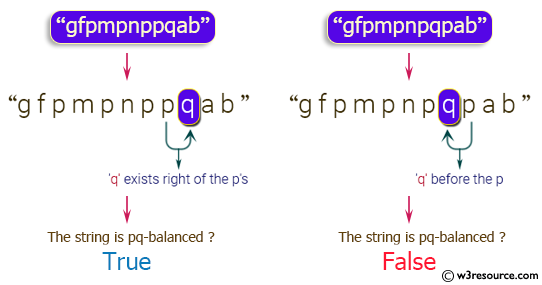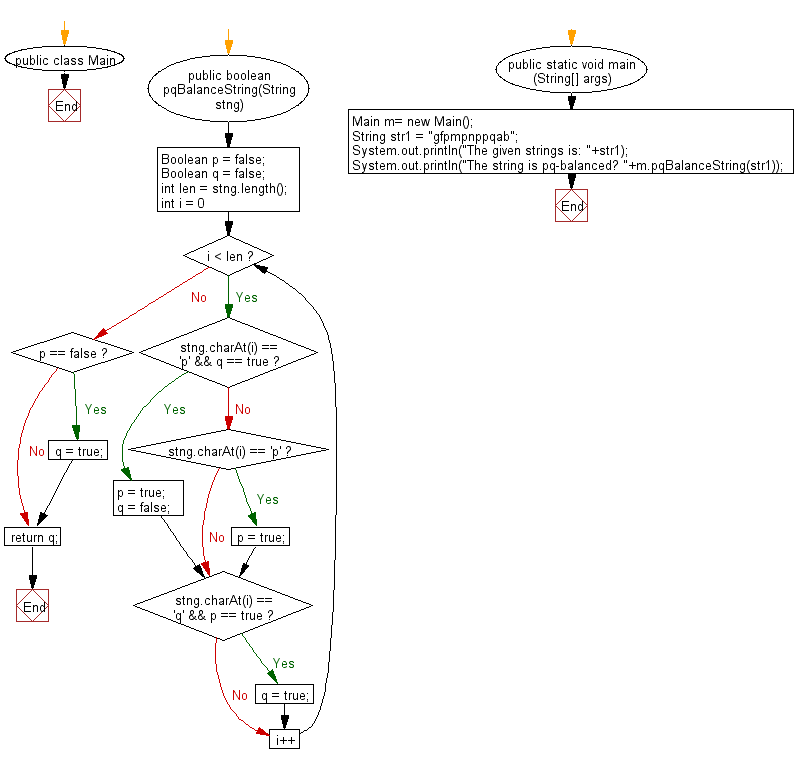﻿ Java exercises: Check whether a string is pq-balanced or not - w3resource# Java String Exercises: Check whether a string is pq-balanced or not

## Java String: Exercise-70 with Solution

Write a Java program to check whether a string is pq-balanced or not. A String is pq-balanced if for all the p's in the string atleast one 'q' must exists right of the p's. But 'q' before the 'p' makes the pq-balanced false.

Sample Solution:

Java Code:

``````import java.util.*;
public class Main
{
public boolean pqBalanceString(String stng)
{
Boolean p = false;
Boolean q = false;
int len = stng.length();
for (int i = 0; i < len; i++)
{
if (stng.charAt(i) == 'p' && q == true)
{
p = true;
q = false;
}
else if (stng.charAt(i) == 'p')
{
p = true;
}
if (stng.charAt(i) == 'q' && p == true)
q = true;
}
if (p == false)
q = true;
return q;
}
public static void main (String[] args)
{
Main m= new Main();
String str1 =  "gfpmpnppqab";
System.out.println("The given strings is: "+str1);
System.out.println("The string is pq-balanced? "+m.pqBalanceString(str1));
}
}
```
```

Sample Output:

```The given strings is: gfpmpnppqab
The string is pq-balanced? true

The given strings is: gfpmpnpqpab
The string is pq-balanced? false
```

Pictorial Presentation:Flowchart:Java Code Editor:

Improve this sample solution and post your code through Disqus

What is the difficulty level of this exercise?

﻿

## Java: Tips of the Day

Directory Content:

Java allows you to get the names of all subdirectories and files in a folder as an array, which can then be sequentially viewed.

```import java.io.*;

public class ListContents {
public static void main(String[] args) {
File file = new File("//home//user//Documents/");
String[] files = file.list();

System.out.println("Listing contents of " + file.getPath());
for(int i=0 ; i < files.length ; i++)
{
System.out.println(files[i]);
}
}
}
```